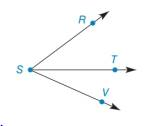Chapter 1.4, Problem 22E### Elementary Geometry for College St...

6th Edition
Daniel C. Alexander + 1 other
ISBN: 9781285195698

#### Solutions

Chapter
Section### Elementary Geometry for College St...

6th Edition
Daniel C. Alexander + 1 other
ISBN: 9781285195698
Textbook Problem
1 views

#Exercises 16 − 24 Given: m ∠ R S T = 2 x 3 m ∠ T S V = x 2 m ∠ R S V = 49 ° Find: x     and m ∠ T S V

To determine

To find:

The value of x and the measure of the angle TSV.

Explanation

Given:

mRST=2x3mTSV=x2mRSV=49°

The given figure is,

Definition:

If a point say M lies in the interior of PQR, then mPQM+mRQM=mPQR.

Calculation:

Consider the figure,

T is the interior point of the angle RSV.

mRST=2x3mTSV=x2mRSV=49°

Use above mentioned angle addition postulate to solve for the value of x

### Still sussing out bartleby?

Check out a sample textbook solution.

See a sample solution

#### The Solution to Your Study Problems

Bartleby provides explanations to thousands of textbook problems written by our experts, many with advanced degrees!

Get Started

#### Sum of Square (SS), variance and standard deviation

Statistics for The Behavioral Sciences (MindTap Course List)

#### Evaluate the integral. 23. 01(1+x)8dx

Single Variable Calculus: Early Transcendentals

#### It does not exist.

Study Guide for Stewart's Multivariable Calculus, 8th

#### Identify and describe the steps of the scientific method.

Research Methods for the Behavioral Sciences (MindTap Course List)